# Count Words

Use TRIM, LEN, SUBSTITUTE and SUMPRODUCT in Excel to count words. First, let's count the total number of words in a cell or range of cells.

1. The TRIM function below returns a string with only regular spaces.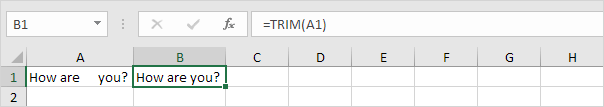2. To get the length of this string, add the LEN function.3. The SUBSTITUTE function below returns a string without spaces.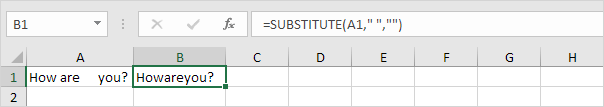4. To get the length of this string, add the LEN function.5. To count the total number of words in cell A1, subtract the length of the string without spaces (formula from step 4) from the length of the string with only regular spaces (formula from step 2) and add 1.Conclusion: to count the total number of words in a cell, simply count the number of spaces and add 1 to this result. 1 space means 2 words, 2 spaces means 3 words, etc.

6. To count the total number of words in the range A1:A2, add the SUMPRODUCT function and replace A1 with A1:A2.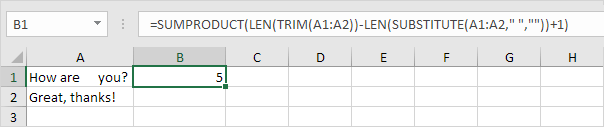Finally, let's count how many times a specific word occurs in a cell or range of cells. This trick is pretty cool.

7. The LEN function below returns the length of the original text in cell A1.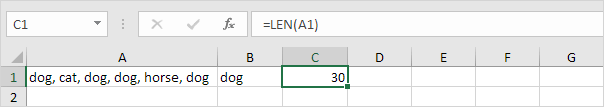8. The SUBSTITUTE function below returns the string without the word "dog".9. The LEN function below returns the length of this string.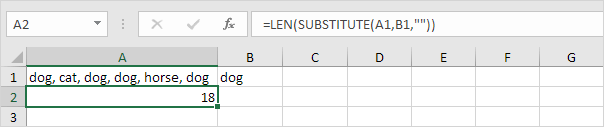10. Subtract the length of the string without the word "dog" (formula from step 9) from the length of the original text in cell A1 (formula from step 7).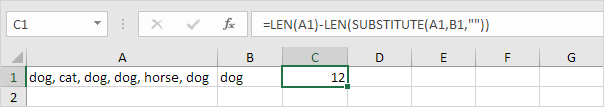Conclusion: after removing the word "dog" from the original text, 12 characters are missing.

11. We know the length of the word "dog" (3), so the word "dog" occurs 12 / 3 = 4 times in cell A1.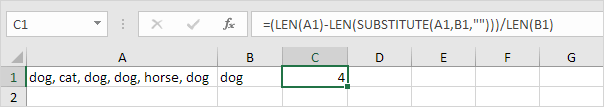12. To count how many times the word "dog" occurs in the range A1:A2, add the SUMPRODUCT function and replace A1 with A1:A2.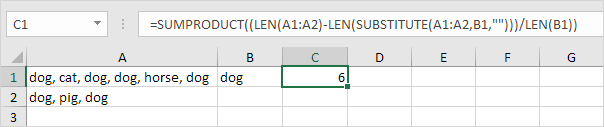13. Use the COUNTIF function in Excel to count the number of cells that contain a specific word.